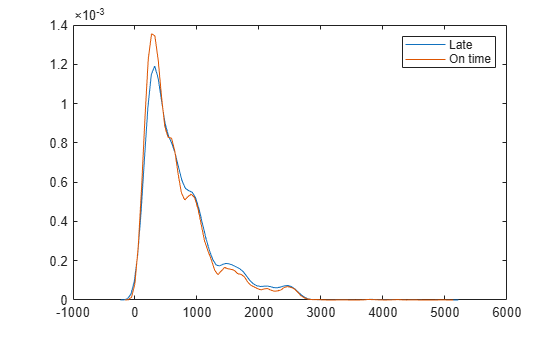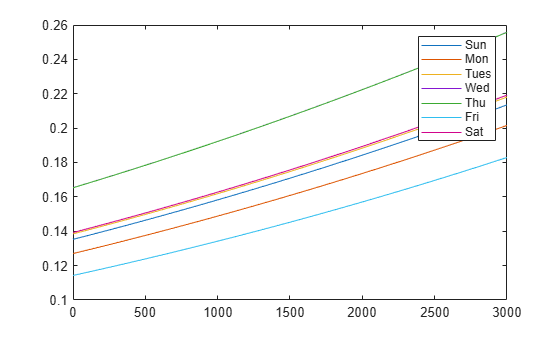# Logistic Regression with Tall Arrays

This example shows how to use logistic regression and other techniques to perform data analysis on tall arrays. Tall arrays represent data that is too large to fit into computer memory.

### Define Execution Environment

When you perform calculations on tall arrays, MATLAB® uses either a parallel pool (default if you have Parallel Computing Toolbox™) or the local MATLAB session. To run the example using the local MATLAB session when you have Parallel Computing Toolbox, change the global execution environment by using the `mapreducer` function.

`mapreducer(0)`

### Get Data into MATLAB

Create a datastore that references the folder location with the data. The data can be contained in a single file, a collection of files, or an entire folder. Treat `'NA'` values as missing data so that `datastore` replaces them with `NaN` values. Select a subset of the variables to work with, and include the name of the airline (`UniqueCarrier`) as a categorical variable. Create a tall table on top of the datastore.

```ds = datastore('airlinesmall.csv'); ds.TreatAsMissing = 'NA'; ds.SelectedVariableNames = {'DayOfWeek','UniqueCarrier',... 'ArrDelay','DepDelay','Distance'}; ds.SelectedFormats{2} = '%C'; tt = tall(ds); tt.DayOfWeek = categorical(tt.DayOfWeek,1:7,... {'Sun','Mon','Tues','Wed','Thu','Fri','Sat'},'Ordinal',true)```
```tt = Mx5 tall table DayOfWeek UniqueCarrier ArrDelay DepDelay Distance _________ _____________ ________ ________ ________ ? ? ? ? ? ? ? ? ? ? ? ? ? ? ? : : : : : : : : : : ```

### Late Flights

Determine the flights that are late by 20 minutes or more by defining a logical variable that is true for a late flight. Add this variable to the tall table of data, noting that it is not yet evaluated. A preview of this variable includes the first few rows.

`tt.LateFlight = tt.ArrDelay>=20`
```tt = Mx6 tall table DayOfWeek UniqueCarrier ArrDelay DepDelay Distance LateFlight _________ _____________ ________ ________ ________ __________ ? ? ? ? ? ? ? ? ? ? ? ? ? ? ? ? ? ? : : : : : : : : : : : : ```

Calculate the mean of `LateFlight` to determine the overall proportion of late flights. Use `gather` to trigger evaluation of the tall array and bring the result into memory.

`m = mean(tt.LateFlight)`
```m = tall double ? ```
`m = gather(m)`
```Evaluating tall expression using the Local MATLAB Session: - Pass 1 of 2: Completed in 0.82 sec - Pass 2 of 2: Completed in 0.92 sec Evaluation completed in 2.2 sec ```
```m = 0.1580 ```

### Late Flights by Carrier

Examine whether certain types of flights tend to be late. First, check to see if certain carriers are more likely to have late flights.

```tt.LateFlight = double(tt.LateFlight); late_by_carrier = gather(grpstats(tt,'UniqueCarrier','mean','DataVar','LateFlight'))```
```Evaluating tall expression using the Local MATLAB Session: - Pass 1 of 1: Completed in 2.3 sec Evaluation completed in 3 sec ```
```late_by_carrier=29×4 table GroupLabel UniqueCarrier GroupCount mean_LateFlight __________ _____________ __________ _______________ {'9E' } 9E 521 0.13436 {'AA' } AA 14930 0.16236 {'AQ' } AQ 154 0.051948 {'AS' } AS 2910 0.16014 {'B6' } B6 806 0.23821 {'CO' } CO 8138 0.16319 {'DH' } DH 696 0.17672 {'DL' } DL 16578 0.15261 {'EA' } EA 920 0.15217 {'EV' } EV 1699 0.21248 {'F9' } F9 335 0.18209 {'FL' } FL 1263 0.19952 {'HA' } HA 273 0.047619 {'HP' } HP 3660 0.13907 {'ML (1)'} ML (1) 69 0.043478 {'MQ' } MQ 3962 0.18778 ⋮ ```

Carriers `B6` and `EV` have higher proportions of late flights. Carriers `AQ`, `ML(1)`, and `HA` have relatively few flights, but lower proportions of them are late.

### Late Flights by Day of Week

Next, check to see if different days of the week tend to have later flights.

`late_by_day = gather(grpstats(tt,'DayOfWeek','mean','DataVar','LateFlight'))`
```Evaluating tall expression using the Local MATLAB Session: - Pass 1 of 1: Completed in 1.4 sec Evaluation completed in 1.6 sec ```
```late_by_day=7×4 table GroupLabel DayOfWeek GroupCount mean_LateFlight __________ _________ __________ _______________ {'Fri' } Fri 15839 0.12899 {'Mon' } Mon 18077 0.14234 {'Sat' } Sat 16958 0.15603 {'Sun' } Sun 18019 0.15117 {'Thu' } Thu 18227 0.18418 {'Tues'} Tues 18163 0.15526 {'Wed' } Wed 18240 0.18399 ```

Wednesdays and Thursdays have the highest proportion of late flights, while Fridays have the lowest proportion.

### Late Flights by Distance

Check to see if longer or shorter flights tend to be late. First, look at the density of the flight distance for flights that are late, and compare that with flights that are on time.

`ksdensity(tt.Distance(tt.LateFlight==1))`
```Evaluating tall expression using the Local MATLAB Session: - Pass 1 of 2: Completed in 1.1 sec - Pass 2 of 2: Completed in 1.3 sec Evaluation completed in 2.9 sec ```
```hold on ksdensity(tt.Distance(tt.LateFlight==0))```
```Evaluating tall expression using the Local MATLAB Session: - Pass 1 of 2: Completed in 1 sec - Pass 2 of 2: Completed in 1.3 sec Evaluation completed in 2.8 sec ```
```hold off legend('Late','On time')```Flight distance does not make a dramatic difference in whether a flight is early or late. However, the density appears to be slightly higher for on-time flights at distances of about 400 miles. The density is also higher for late flights at distances of about 2000 miles. Calculate some simple descriptive statistics for the late and on-time flights.

`late_by_distance = gather(grpstats(tt,'LateFlight',{'mean' 'std'},'DataVar','Distance'))`
```Evaluating tall expression using the Local MATLAB Session: - Pass 1 of 1: Completed in 1.2 sec Evaluation completed in 1.6 sec ```
```late_by_distance=2×5 table GroupLabel LateFlight GroupCount mean_Distance std_Distance __________ __________ __________ _____________ ____________ {'0'} 0 1.04e+05 693.14 544.75 {'1'} 1 19519 750.24 574.12 ```

Late flights are about 60 miles longer on average, although this value makes up only a small portion of the standard deviation of the distance values.

### Logistic Regression Model

Build a model for the probability of a late flight, using both continuous variables (such as `Distance`) and categorical variables (such as `DayOfWeek`) to predict the probabilities. This model can help to determine if the previous results observed for each predictor individually also hold true when you consider them together.

`glm = fitglm(tt,'LateFlight~Distance+DayOfWeek','Distribution','binomial')`
```Iteration : 0% completed Iteration : 100% completed Iteration : 0% completed Iteration : 100% completed Iteration : 0% completed Iteration : 100% completed Iteration : 0% completed Iteration : 100% completed Iteration : 0% completed Iteration : 100% completed ```
```glm = Compact generalized linear regression model: logit(LateFlight) ~ 1 + DayOfWeek + Distance Distribution = Binomial Estimated Coefficients: Estimate SE tStat pValue __________ __________ _______ __________ (Intercept) -1.855 0.023052 -80.469 0 DayOfWeek_Mon -0.072603 0.029798 -2.4365 0.01483 DayOfWeek_Tues 0.026909 0.029239 0.92029 0.35742 DayOfWeek_Wed 0.2359 0.028276 8.343 7.2452e-17 DayOfWeek_Thu 0.23569 0.028282 8.3338 7.8286e-17 DayOfWeek_Fri -0.19285 0.031583 -6.106 1.0213e-09 DayOfWeek_Sat 0.033542 0.029702 1.1293 0.25879 Distance 0.00018373 1.3507e-05 13.602 3.8741e-42 123319 observations, 123311 error degrees of freedom Dispersion: 1 Chi^2-statistic vs. constant model: 504, p-value = 8.74e-105 ```

The model confirms that the previously observed conclusions hold true here as well:

• The Wednesday and Thursday coefficients are positive, indicating a higher probability of a late flight on those days. The Friday coefficient is negative, indicating a lower probability.

• The Distance coefficient is positive, indicating that longer flights have a higher probability of being late.

All of these coefficients have very small p-values. This is common with data sets that have many observations, since one can reliably estimate small effects with large amounts of data. In fact, the uncertainty in the model is larger than the uncertainty in the estimates for the parameters in the model.

### Prediction with Model

Predict the probability of a late flight for each day of the week, and for distances ranging from 0 to 3000 miles. Create a table to hold the predictor values by indexing the first 100 rows in the original table `tt`.

`x = gather(tt(1:100,{'Distance' 'DayOfWeek'}));`
```Evaluating tall expression using the Local MATLAB Session: - Pass 1 of 1: Completed in 0.22 sec Evaluation completed in 0.37 sec ```
```x.Distance = linspace(0,3000)'; x.DayOfWeek(:) = 'Sun'; plot(x.Distance,predict(glm,x)); days = {'Sun' 'Mon' 'Tues' 'Wed' 'Thu' 'Fri' 'Sat'}; hold on for j=2:length(days) x.DayOfWeek(:) = days{j}; plot(x.Distance,predict(glm,x)); end legend(days)```According to this model, a Wednesday or Thursday flight of 500 miles has the same probability of being late, about 18%, as a Friday flight of about 3000 miles.

Since these probabilities are all much less than 50%, the model is unlikely to predict that any given flight will be late using this information. Investigate the model more by focusing on the flights for which the model predicts a probability of 20% or more of being late, and compare that to the actual results.

`C = gather(crosstab(tt.LateFlight,predict(glm,tt)>.20))`
```Evaluating tall expression using the Local MATLAB Session: - Pass 1 of 1: Completed in 1.1 sec Evaluation completed in 1.2 sec ```
```C = 2×2 99613 4391 18394 1125 ```

Among the flights predicted to have a 20% or higher probability of being late, about 20% were late `1125/(1125 + 4391)`. Among the remainder, less than 16% were late `18394/(18394 + 99613)`.